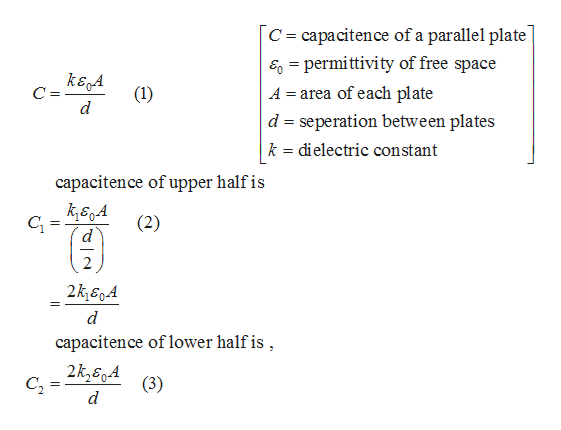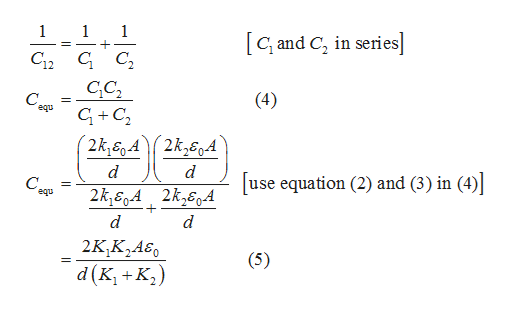# The figure shows a parallel-plate capacitor with a plate area A = 2.52 cm2 and plate separation d = 7.95 mm. The bottom half of the gap is filled with material of dielectric constant κ1 = 13.7; the top half is filled with material of dielectric constant κ2 = 12.2. What is the capacitance?

Question
515 views

The figure shows a parallel-plate capacitor with a plate area A = 2.52 cm2 and plate separation d = 7.95 mm. The bottom half of the gap is filled with material of dielectric constant κ1 = 13.7; the top half is filled with material of dielectric constant κ2 = 12.2. What is the capacitance?

check_circle

Step 1

When capacitors are connected in series, the total capacitance is less than any one of the series capacitors’ individual capacitance. In this case, the upper and the lower capacitors are arranged in series so that equivalent capacitance is given by equation (4).

Step 2help_outlineImage TranscriptioncloseC capacitence of a parallel plate E permittivity of free space A area of each plate = ksA d d seperation between plates k dielectric constant capacitence of upper half is C (2) 2k&A d capacitence of lower half is 2k360A C2 (3) d fullscreen
Step 3help_outlineImage Transcriptionclose1 1 + 1 [Gand C in series] С с, C12 CC2 (4) equ GC2 2k,sgA 2k360A C. 2k,SA 2k,6 use equation (2) and (3) in (4) equ d d 2KK2A60 (5) ( p fullscreen

### Want to see the full answer?

See Solution

#### Want to see this answer and more?

Solutions are written by subject experts who are available 24/7. Questions are typically answered within 1 hour.*

See Solution
*Response times may vary by subject and question.
Tagged in

### Semiconductors